Digital Electronics: Assignment

# Digital Electronics: Assignment Notes | Study Solid State Physics, Devices & Electronics - Physics

## Document Description: Digital Electronics: Assignment for Physics 2022 is part of Digital Electronics for Solid State Physics, Devices & Electronics preparation. The notes and questions for Digital Electronics: Assignment have been prepared according to the Physics exam syllabus. Information about Digital Electronics: Assignment covers topics like and Digital Electronics: Assignment Example, for Physics 2022 Exam. Find important definitions, questions, notes, meanings, examples, exercises and tests below for Digital Electronics: Assignment.

Introduction of Digital Electronics: Assignment in English is available as part of our Solid State Physics, Devices & Electronics for Physics & Digital Electronics: Assignment in Hindi for Solid State Physics, Devices & Electronics course. Download more important topics related with Digital Electronics, notes, lectures and mock test series for Physics Exam by signing up for free. Physics: Digital Electronics: Assignment Notes | Study Solid State Physics, Devices & Electronics - Physics
 1 Crore+ students have signed up on EduRev. Have you?

Q.1. (a) Convert (1101101)2 to decimal number.
(b) Convert (11101.011)2 to decimal number.
(c) Convert decimal number 12 to binary number.
(d) Convert decimal number 0.3125 to binary number.
(e) Convert octal number (2374)8 to decimal number.
(f) Convert octal number (0.325)8 to decimal number.
(g) Convert decimal number 359 to octal number.
(h) Convert each of the following octal numbers to binary numbers.
(i) 138 (ii) 258 (iii) 478 (iv) 1708 (v) 7528 (vi) 52768 (vii) 37.128
(i) Represent each of the following binary numbers by its octal equivalent:
(a) 1101012 (b) 1011110012 (c) 10111001102 (d) 1001101.10112
(j) Convert binary numbers to hexadecimal number.
(a) 11001010010101112 (b) 1111110001011010012 (c) 1110011000.1112
(k) Determine the binary numbers for the following hexadecimal numbers:
(a) 10A416 (b) CF8316 (c) 974216 (d) D2E.816
(l) Convert the following hexadecimal numbers to decimal.
(a) 1C16 (b) A8516
(m) Convert (a) E 516 and (b) B 2F816 to decimal.
(n) Convert 65010 to hexadecimal by repeated division by 1610.
(o) Find 1's compliment and 2's compliments of binary number (10101), (10111) and (111100).

(a)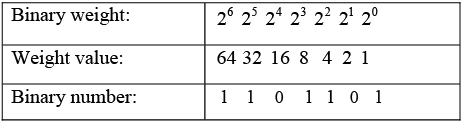1 x 64 +1 x 32 + 0 x 16 +1 x 8 +1 x 4 + 0 x 2 +1 x 1 = 64 + 32 + 0 + 8 + 4 + 0 +1 = 10910
(b) (16 + 8 + 4 + 1) . ( 0.25 + 0.125) = 29.37510
(c)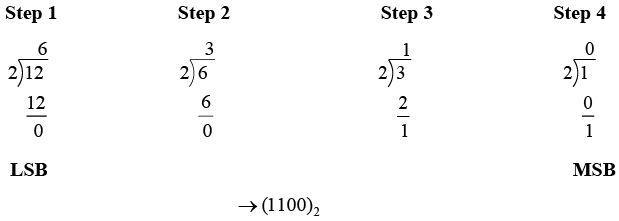(d) Step 1 (0.3125 x 2 = 0.625 → carry = 0 ( MSB))
Step 2 (0.625 x 2 = 1.25 → carry = 1
Step 3 (0.25 x 2 = 0.50 → carry = 0
Step 4 (0.50 x 2 = 1.00 → carry = 1 ( LSB) → (0.0101)2)
(e)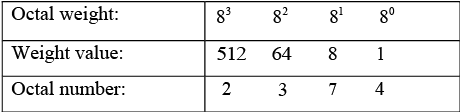2 x 512 + 3 x 64 + 7 x 8 + 4 x 1 = 127610
(f)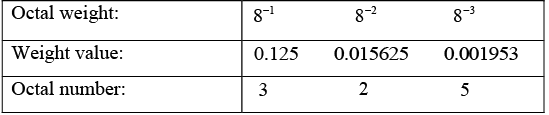3 x 0.125 + 2 x 0.015625 + 5 x 0.001953 = 0.41601510
(g)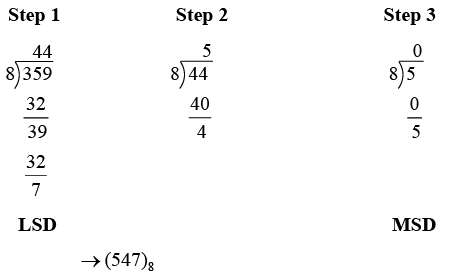(h)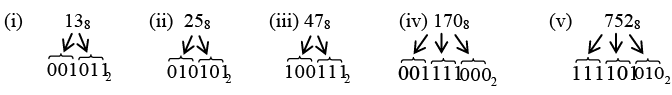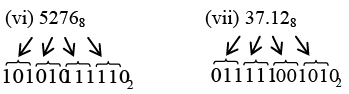(i)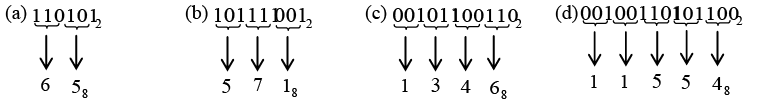(j)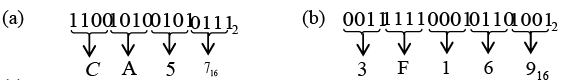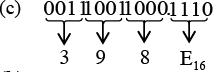(k)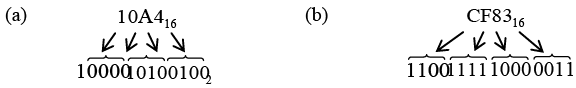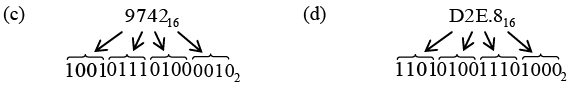(l)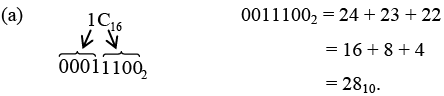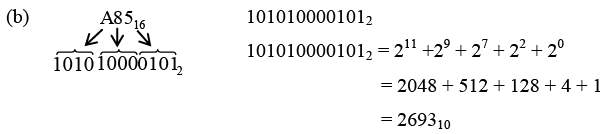(m) (a) E516 = E x 16 + 5 x 1 = 14 x 16 +5 x 1 = 224 +5 = 22910
(b) B2F816 = B x 4096 + 2 x 256 + F x16 + 8 x 1
= 11 x 4096 + 2 x 256 + 15 x 16 + 8 x 1
= 45056 + 512 + 240 + 8 = 4581610
(n)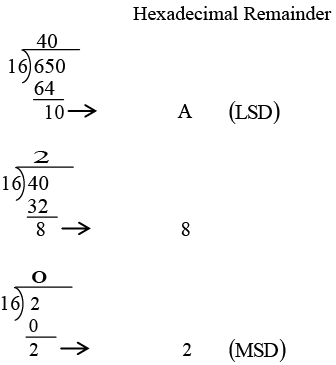Therefore, 65010 = 28A16.
(o)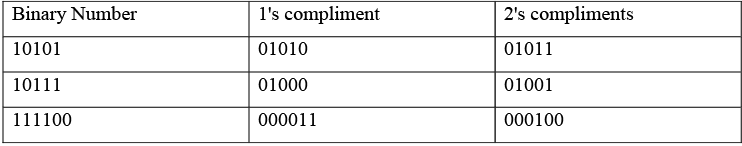Q.2. For the logic circuit shown in figure, the input conditions (A, B, C) are given. In each case find output X.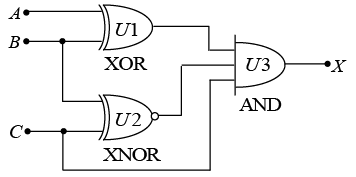(a) 1,0,1
(b) 0,0,1
(c) 1,1,1
(d) 0,1,1

XOR is inequality comparator and XNOR is equality comparator. In AND gate output will be high when all the input is 1.
(a) (A, B,C) = (1, 0,1) ; U1 = 1, U2 = 0 ⇒ U3 = 0
(b) (A, B,C) = (0,0,1) ; U1 = 0 , U2 = 0 ⇒ U3 = 0
(c) (A, B, C) = (1,1,1) ; U1 = 0 , U2 = 1 ⇒ U3 = 0
(d) (A, B, C) = ( 0,1,1) ; U1 = 1 , U2 = 1 ⇒ U3 = 1

Q.3. In each case find output Boolean expression.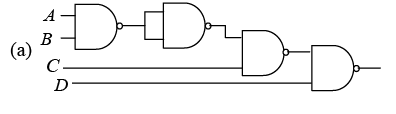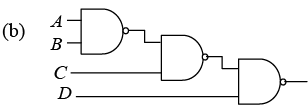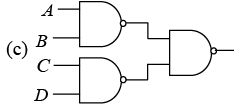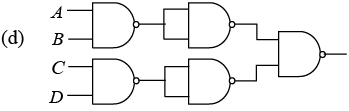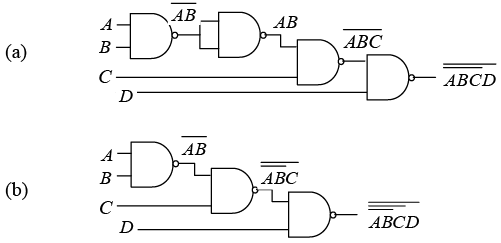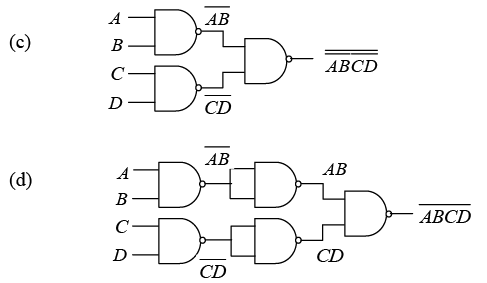Q.4. Find the output of combinational circuit shown in figure below.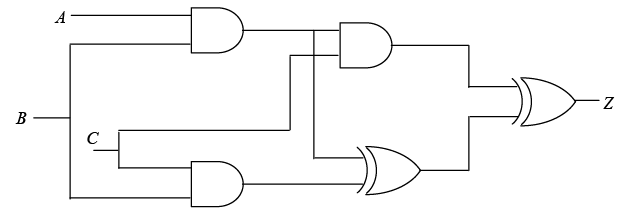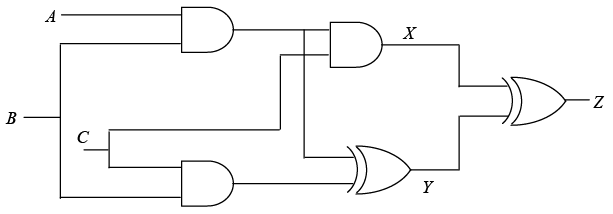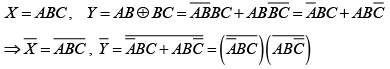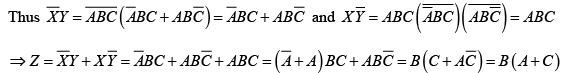Q.5. Consider the digital circuit shown below in which the input C is always low (0).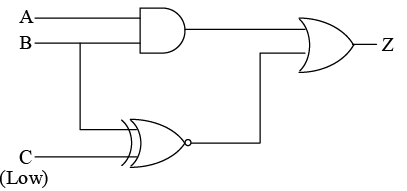Write the truth table for the circuit shown in figure.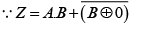The truth table for the circuit can be written as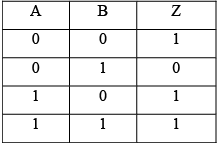Q.6. Write the truth table for the circuit shown in figure.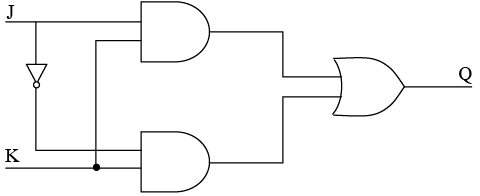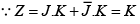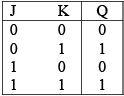Q.7. Using basic gates  implement the truth table?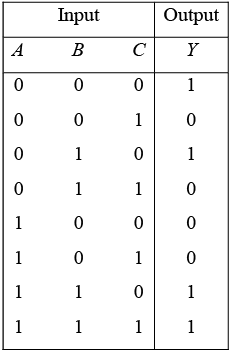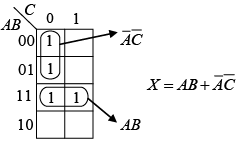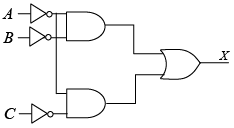Q.8. To implement the function f = (x + z)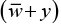how many two input NOR gate would require.

f = ( x + z )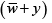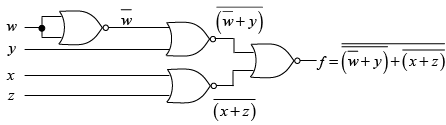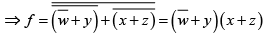So, 4 NOR gate is required.

Q.9. Find the minimized expression for Boolean function
f(a, b, c, d) = ∑m (0, 2, 3, 5, 7, 8, 9,10,11) + ∑dc (4,15)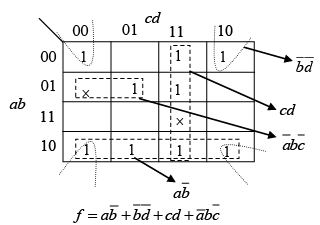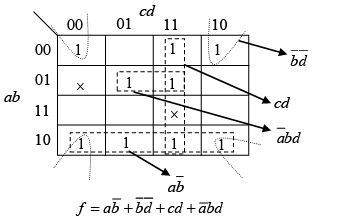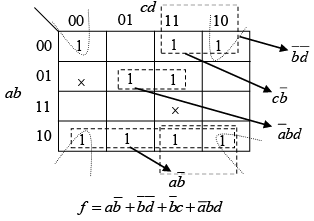Q.10. A function with don’t cares is as follows:
g (X , Y , Z) =∑m (5, 6)+∑dc (1, 2, 4)
For above function consider following expression
(1)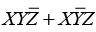(2)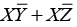(3)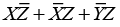(4)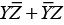Which of the above expressions are solution for g.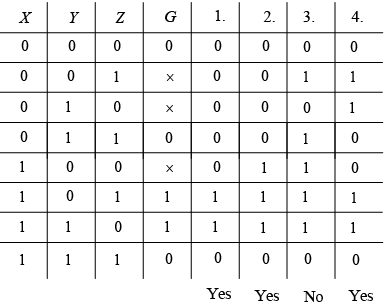So expressions (1), (2) and (4) are solutions for g.

The document Digital Electronics: Assignment Notes | Study Solid State Physics, Devices & Electronics - Physics is a part of the Physics Course Solid State Physics, Devices & Electronics.
All you need of Physics at this link: Physics

## Solid State Physics, Devices & Electronics

91 videos|21 docs|25 tests
 Use Code STAYHOME200 and get INR 200 additional OFF

## Solid State Physics, Devices & Electronics

91 videos|21 docs|25 tests

Track your progress, build streaks, highlight & save important lessons and more!

,

,

,

,

,

,

,

,

,

,

,

,

,

,

,

,

,

,

,

,

,

,

,

,

;